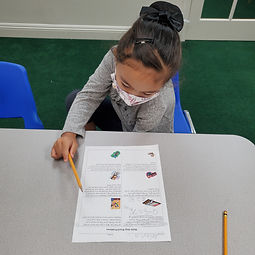## Ms. Raejean

### Target 1​

###### Lesson Type:

Review

Number Operation

:

Fractional Numbers

Make equivalent values of money.

###### 1:

Represent a given amount of money in different ways.

###### 2:

Show how different combinations of coins equal the same amount of money.

3rd

###### Vocabulary:

Money, Quarters, Dimes, Nickels, Pennies, Combinations, Cents (¢)

Activities:

Students found 2 different ways to make a given amount of money . Students used different combinations of coins. Ex. 71¢ can be made with 2 quarters, 2 dimes, and 1 penny OR 1 quarter, 4 dimes, 1 nickel, and 1 penny. Students counted the money aloud. We worked on making "friendly" amounts when adding the coins. Ex. 25¢ + 5¢ + 10¢ + 10¢ + 10¢ + 10¢ + 1¢ = 71¢### Home Exploration

###### Guiding Questions:## Absent Students:

### Target 2

:

###### 1:

Recognize that a time on the hour will have the minute hand on the 12 on an analog clock and 00 on the digital clock.

###### 2:

Recognize that a time on the half-hour will have the minute hand on the 6 on an analog clock and 30 on the digital clock.

###### 3:

Learn the meaning of time expressions such as half-past, quarter-past, and quarter-to.

3rd

###### Vocabulary:

Analog, Hour, Minutes, Half Past, Quarter To, Quarter Past

Activities:

We reviewed the Hour and Minute hands on the clock. We also discussed how to read the numbers of the clock. When the hour hand is on a number, it points to the hour. When the minute hand points to a number, we count by 5.

The phrases Half Past, Quarter To, and Quarter Past were introduced. We attempted to practice using the phrases to indicate the time. We will revisit this at a later time.### Home Exploration

###### Guiding Questions:### Target 3

:

###### 1:

Identify the correct arithmetic processes based on the information presented in word problems.

###### 2:

Find the key words in word problems that indicate the correct arithmetic process to use.

###### 3:

Find and use the needed information in a word problem in order to solve.

3rd

###### Vocabulary:

Activities:

Students solved multi-step word problems. We read the problems at least 2 times to make sense of what the problem is asking (underline) and the important information that is needed to solve it (circle). Students wrote equations and determined a strategy to solve the problem. Ex. Step 1: 12 - 5 = 7. Step 2: 7 x \$4. Student solved the problem by first finding 12 x \$4 by skip counting, then crossing out the last 5 jumps. The problems provided an opportunity to review multiplication and division. Ex. After adding 24 boys and 39 girls, students had to divide them into groups of 9. Students used a hundreds chart to skip count by 9 to find out how many groups could be made.### Home Exploration